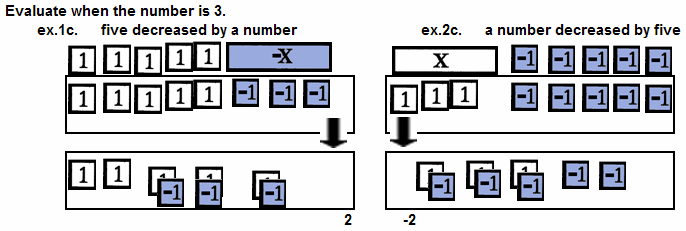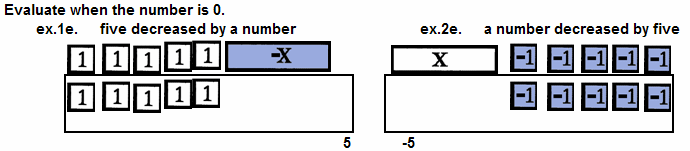The Visual / Auditory / Symbolic / Kinesthetic Approach to Algebra

 "Five Decreased by a Number" Vs, "A Number Decreased by Five"

Many beginning algebra students don't recognize a difference between "five decreased by a number" and "a number decreased by five."

They remember "five increased by a number" is equivalent to "a number increased by five," but forget that SUBTRACTION IS NOT COMMUTATIVE. In subtraction order does count. The expressions are not the same.

5 - x         does not equal         x - 5

Writing the expressions first on paper with pencil, really doesn't emphasize the difference. The expressions still look too similar.

In the nonmanipulative math class, repeated evaluation with different values of the variable makes the difference clear but it is not a memorable distinction for the weak student.

In the nonmanipulative math class, students are told, "You just have to remember which comes first."

In a Term Tile empowered class, the Tiles make the distinctions clear and memorable, the repeated evaluation reinforces the difference even for the weak student.Before beginning the exercises below, recall two ideas.
• When evaluating "the opposite of a number," you must replace the opposite-of-a-number- tile, -x, with the opposite of the number.

• Manipulatives are designed to be outgrown. Encourage mental replacement as soon as it is practical.Consider.

Consider the resuslts found in the above examples and generalize.

 five decreased by a number a number decreased by five Evaluate when 5 - x x - 5 the number is 3. 2 -2 the number is -2. 7 -7 the number is 0. 5 -5 the number is -2x. 2x + 5 -2x - 5 the number is 5. 0 0

LOOKS LIKE:BUT IS IT?

"Five decreased by a number" is the OPPOSITE of "a number decreased by five," but one must solve the equation 5 - x = -(x - 5) first and understand the solution. This is left for the reader. See the technique used below.

Verify or Disprove.
 ex. I. "Five decreased by a number is equal to a number decreased by five" is true when the number is 5.    The statement is true.     See examples 1g. and 2g.   Each expression is equal to 0. ex. J. Five decreased by a number is equal to a number decreased by five.    This statement can not be verified or disproved with a simple evaluation of exresssions. It requires solving an equation. See Solving Equations and Inequalities.The graph at the right extends the examination of the statement "Five decreased by a number is equal to a number decreased by five" beyond manipulatives. It shows each expression represented by the appropriate line and the fact that lines cross ONLY when x is 5, the point of intersection, the solution to the equation."The Other Number If The Sum Of Two Numbers Is Five"

Suppose you have 5 dollars and spend some of it. How much is left?If you replies with good old 5 - x, 5 decreased by a number, you are correct. Its opposite x - 5, a number decreased by 5, just doesn't do the job.

The same format will, of course, work for all expressions of this type. But, still the know-value-decreased-by-an-unknown-amout is a difficult idea for many to master.

Represent.
ex. K.     The are 4 coins.
Some are dimes and the rest are quarters. How many are quarters?www.termtiles.com, Unit 8   © 2008, A. Azzolino